## General Chemistry

Quantum numbers tell us the energy level, the number and the type of orbitals, and the spin of the electron. Collectively, they all describe the electron configurations.

There are four quantum numbers that we are going to discuss:

• The Principal Quantum Number (n) – indicates the main energy level of orbitals and electrons
• The Angular Momentum Quantum Number (l) – indicates the energy sublevel, which is given by the type of the orbital (s, p, d, f)
• The Magnetic Quantum Number, (ml) – indicates the specific orbital within the energy sublevel
• The Electron Spin Quantum Number (ms) – shows the direction of the electron spin

So, one can visualize the information conveyed by quantum numbers getting more specific as we go from the principal quantum number to the spin quantum number: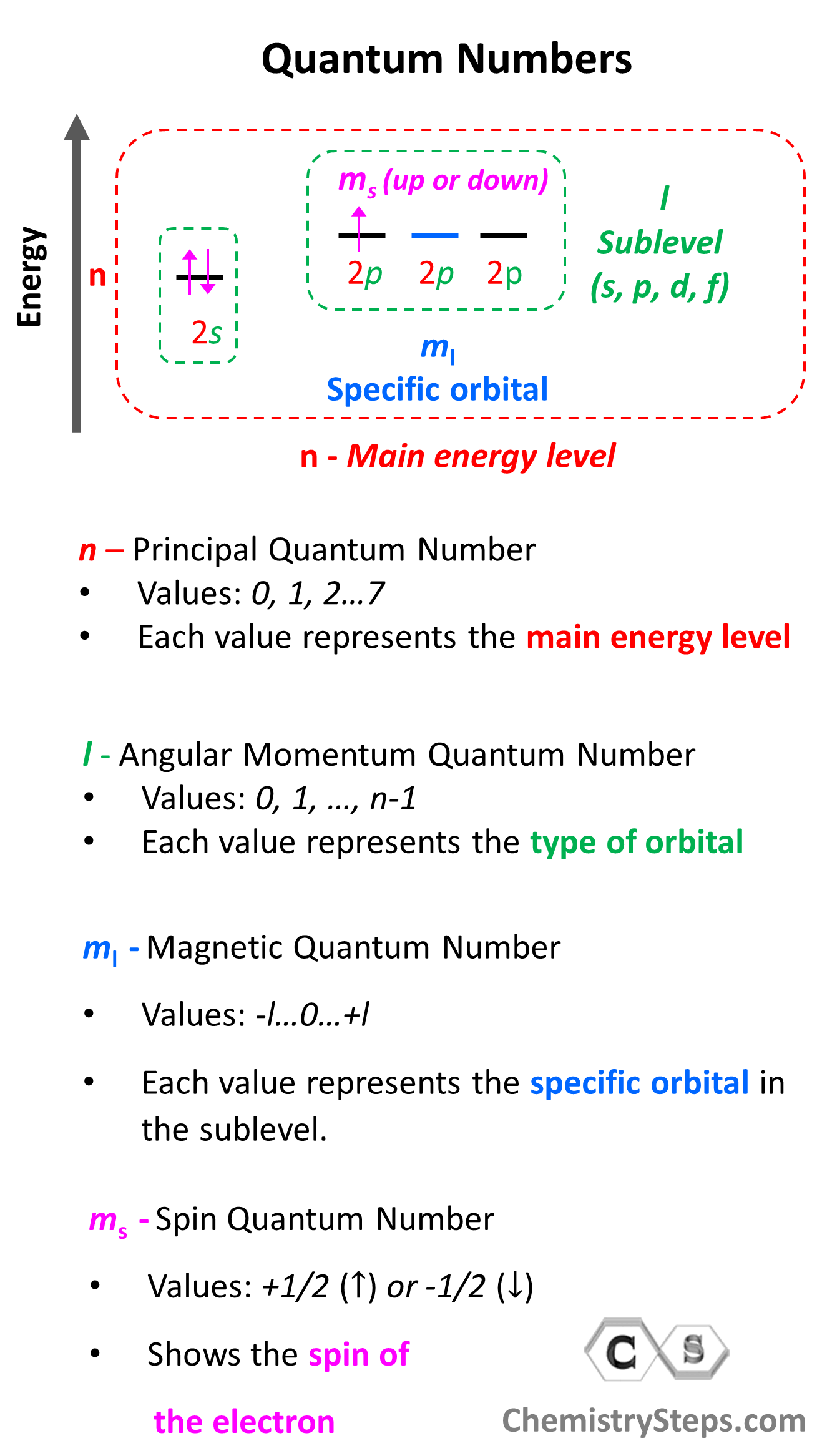Let’s now discuss the quantum numbers one by one in more detail.

# Principal Quantum Number (n)

What orbitals a given atom has, and in which ones the electrons are located, depends on the energy level of the atom. Remember, the energy level of the atom is given by the principal quantum number, n which can easily be determined based on the period (row) the atom is located in the periodical table.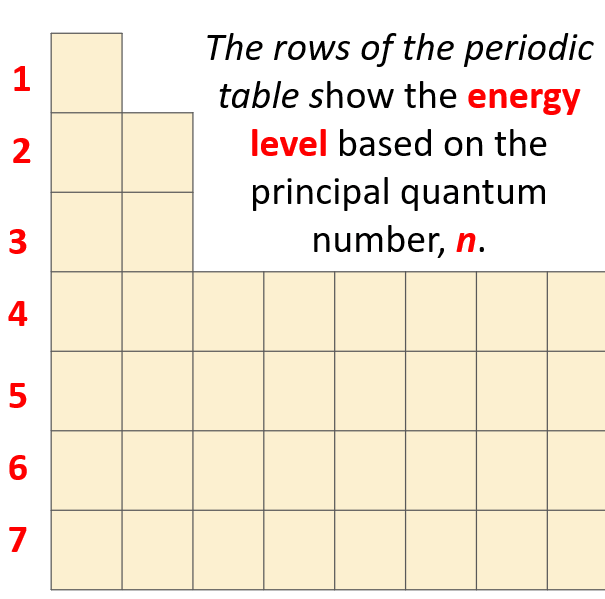This is what we discussed about the Bohr model of the hydrogen atom. There are orbits with fixed radii each associated with discrete energy, and this is described by the principal quantum number n.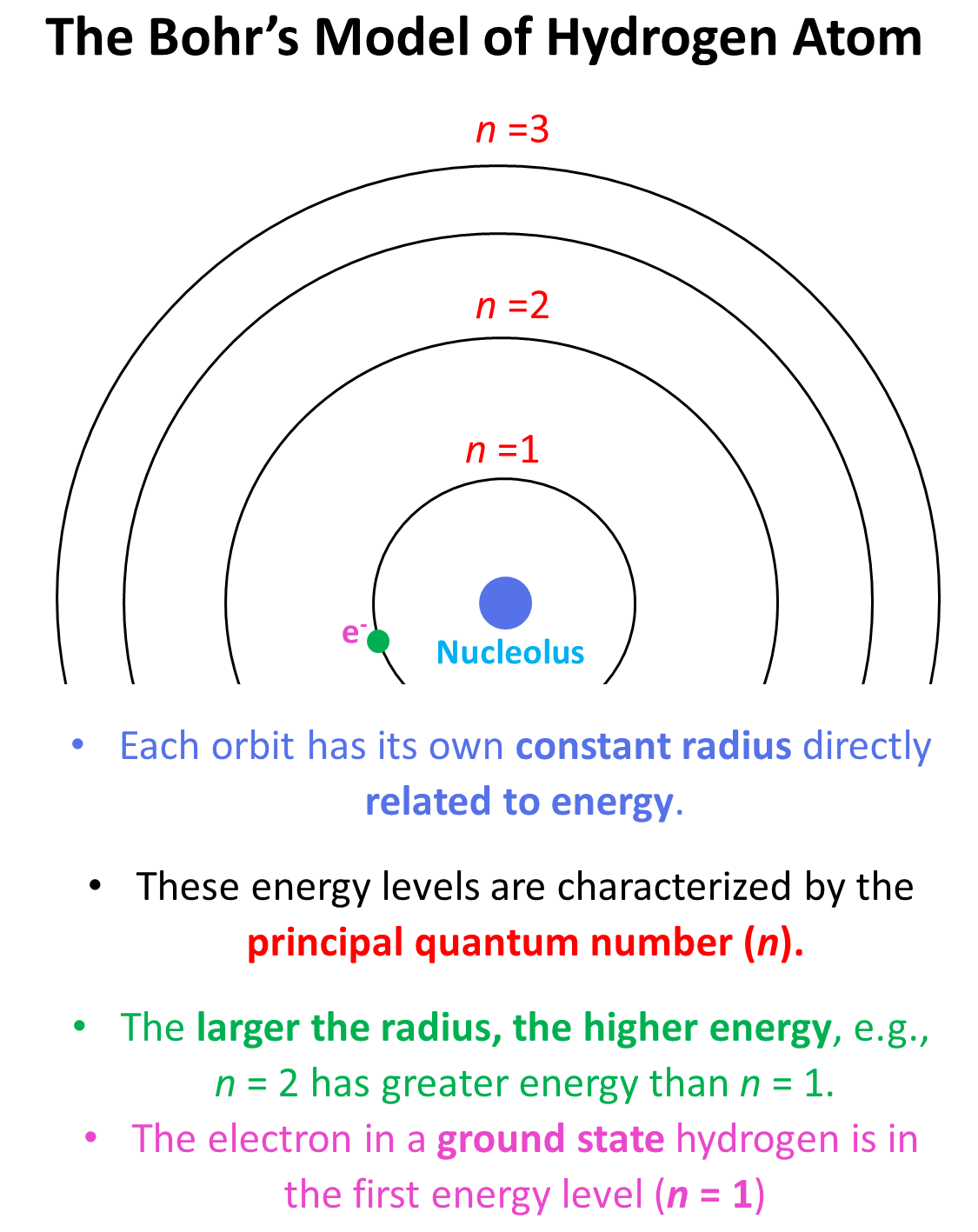Remember, there are four types of atomic orbitals – spd, and f. Each orbital has a characteristic shape shown below: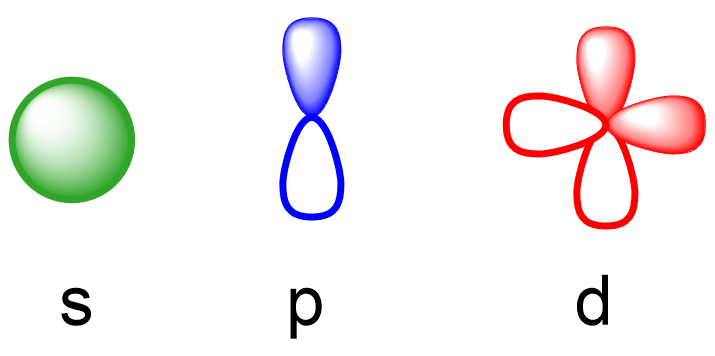S orbitals have a spherical shape, p orbitals are dumbbell-shaped, d orbitals are shaped like a cloverleaf, and f orbitals are characterized by more complex shapes. You can also look up more detailed images for the shapes and orientation of atomic orbitals in your textbook.

The number of types of orbitals matches the energy level: the first energy level has only 1 (s) orbital, the second has two types – s and p, the third has threes, p, and d, and the fourth level has all four types of orbitals – s, p, d, and f.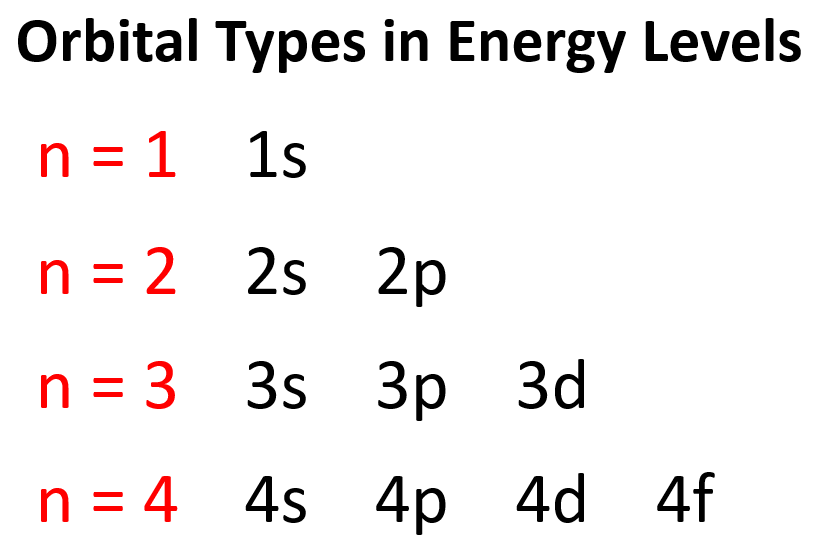So, far we have talked about the main energy level. However, you should know, aside from the first energy level, all the others have sublevels, and these are the types of orbitals that we have talked about – s, p, d, and f.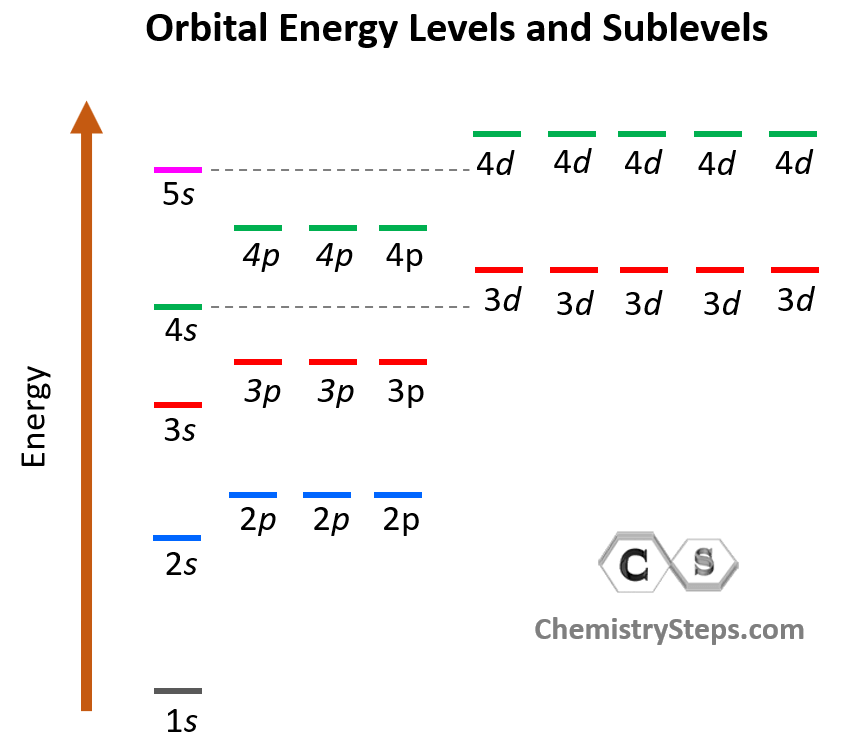Notice again that within the same principal level, orbitals with a lower value of l have lower energy (E) and therefore, are filled first. So, for a given value of n:

(orbital) < orbital) < (orbital) < orbital)

Now, a few important things about the orbitals and their electron capacity. First, remember that each orbital, whether it is s, p, d, or f can accommodate two electrons at most.

We can see this in orbital diagrams where the orbitals are shown as boxes and electrons as arrows, we never put more than two arrows in the box. For example, boron has two electrons in each s orbital of the first and second levels, and one electron in the p sublevel.# Angular Momentum Quantum Number (l)

So, how do we know what sublevels (types of orbitals) a given energy level has? This is determined by the Angular Momentum Quantum Number (l). It takes values of 0, 1, … n-1. For example, for the second energy level, n = 2, and therefore, l = 0, 1 , so it can have two values, and therefore, the second energy level has two sublevels – s (l = 0) and p (l = 1).

Let’s put the orbitals corresponding to each value of l in a diagram as well: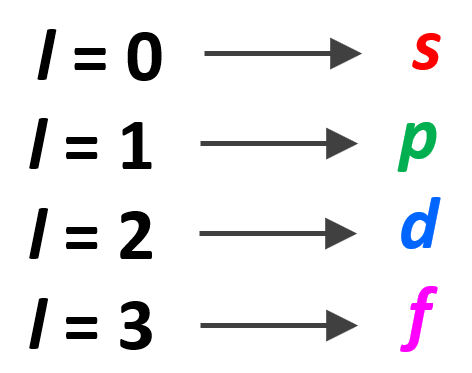Example: Identify the main energy level, the sublevel, and the maximum number of electrons that can have the following quantum numbers: n = 3, l = 2. Draw the orbital diagram to explain your answer.

Solution: The main energy level is given by the principal quantum number (n), so this is an orbital in the 3rd energy level. For the sublevel (the type of the orbital), we need to look at the angular momentum quantum number, l, and when l = 2, we have d orbitals. Therefore, this combination represents the 3d sublevel: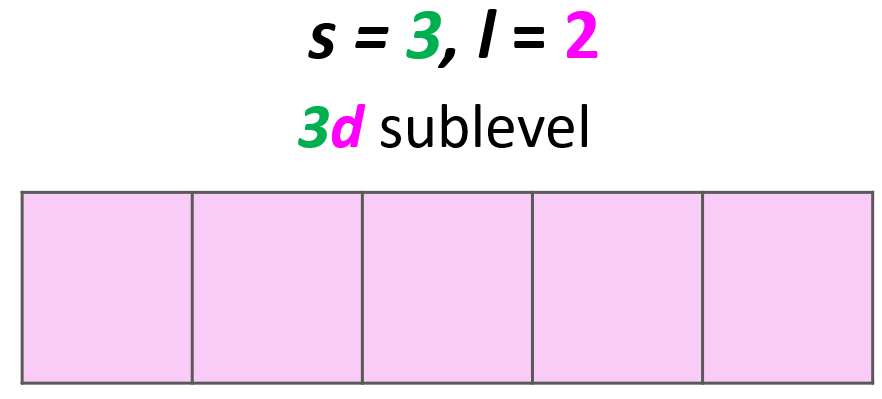Each d sublevel has five orbitals, and because each orbital can accommodate two electrons at most, the maximum number of electrons will be 10.# Magnetic Quantum Number, ml

The next quantum number is the Magnetic Quantum Number, ml which shows the number of orbitals in the sublevel. It takes values from l to +l including the zero and all the integers. For example, when l = 2, we have d orbitals, and because ml = -2, -1, 0, +1, +2, there are 5 orbitals in each sublevel.

The summary of quantum numbers including their meaning and values is given in the diagram below: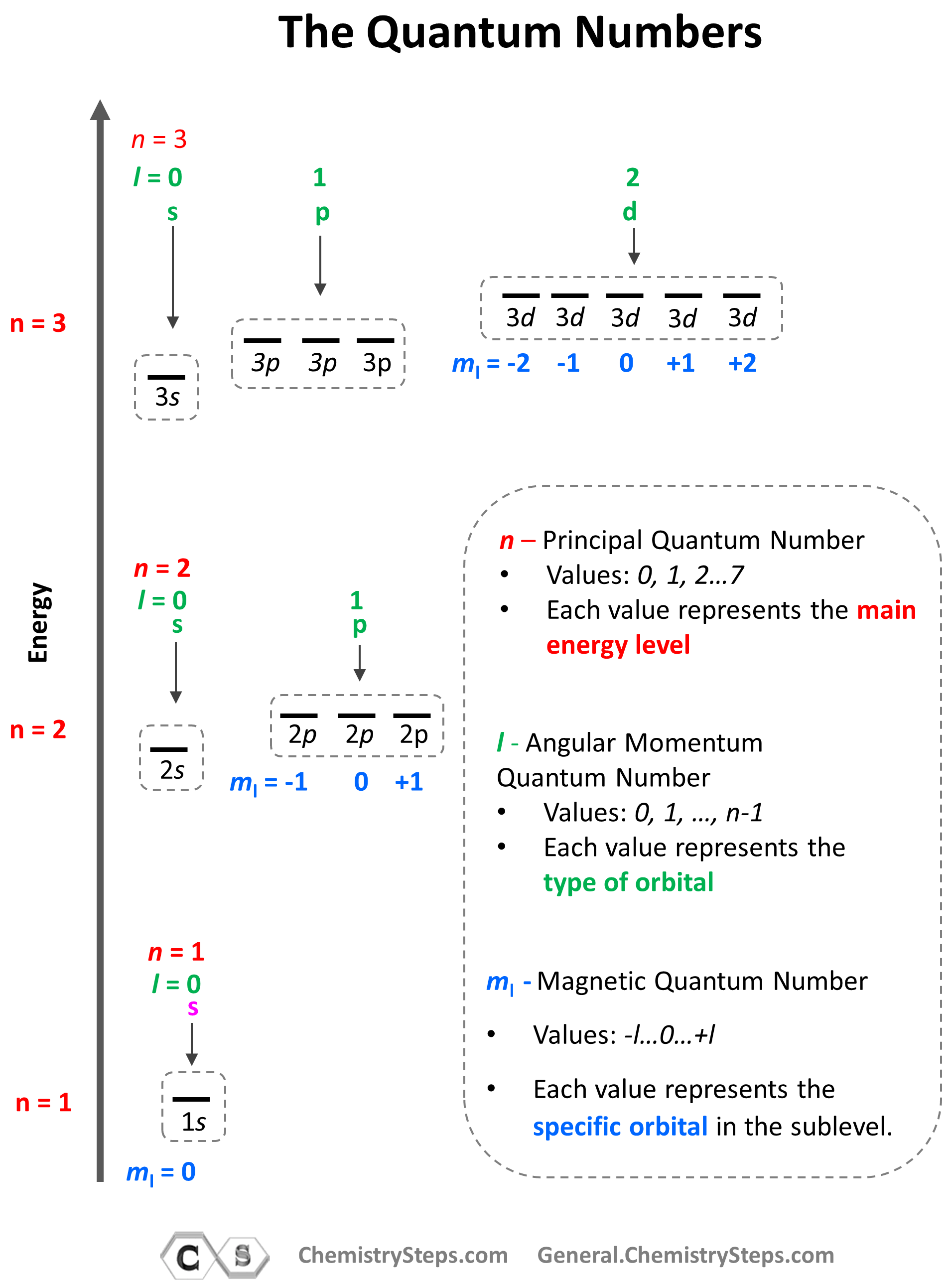Based on the values of ml (-l … 0 … +l), there can only be s orbital in the given energy level, p orbitalsd orbitals, and f orbitals. And because each orbital can only take a maximum of two electrons, there can only be a maximum of two electrons in any s sublevel6 electrons in the p subshell10 in the d, and 14 in the f sublevel.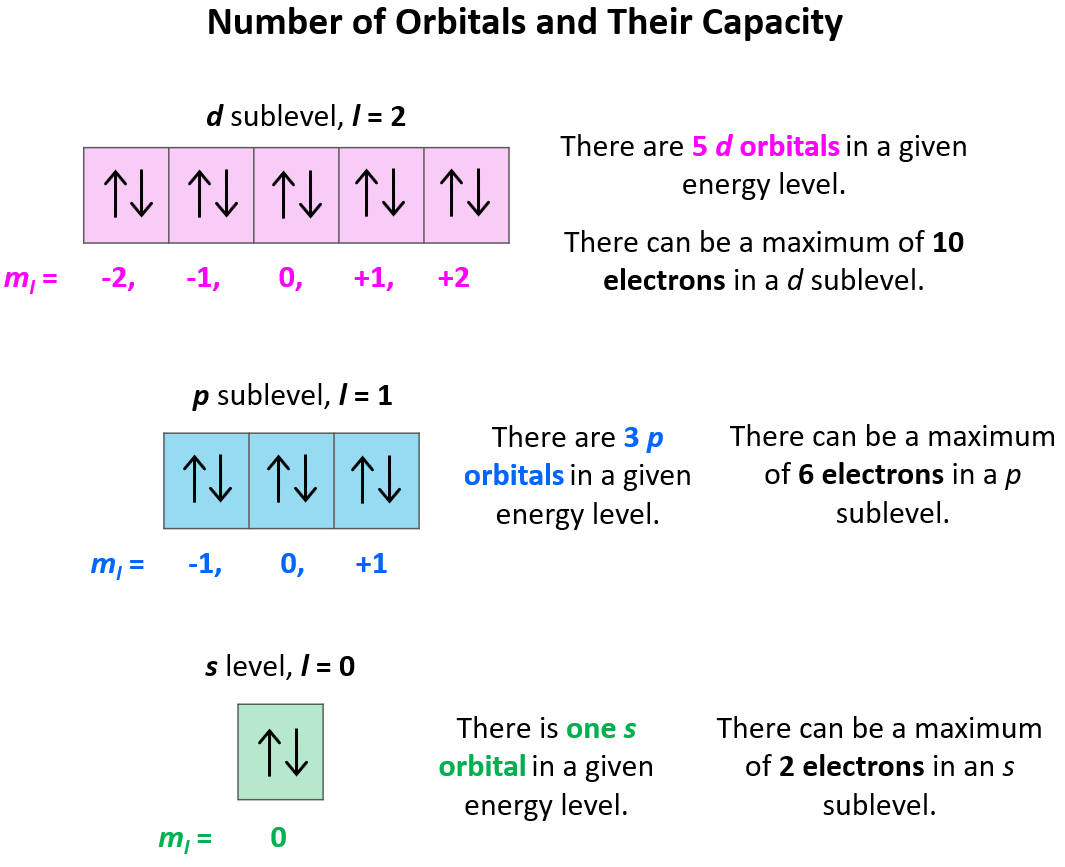We have now covered the first three quantum numbers, and these are sufficient to identify any specific orbital and electron. The only thing we cannot figure out based on these is the spin of a given electron which we will discuss in the next section.

For example, which orbital is indicated by the following set of quantum numbers: n = 3, l = 2, ml = 0?

Starting with the principal quantum number, we know that it is an orbital in the 3rd energy level. l = 2, indicates a d orbital, and ml = 0 indicates the middle one of the five d orbitals.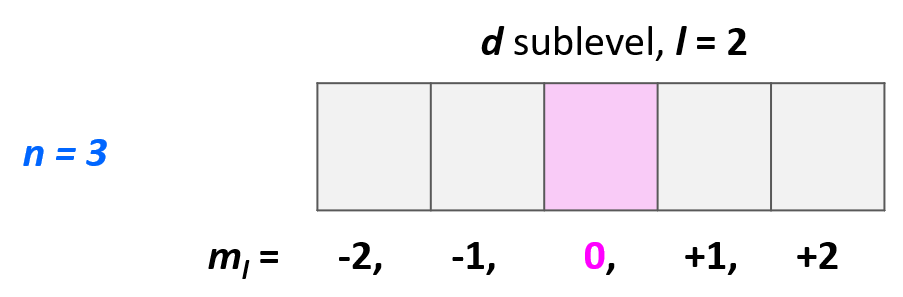# The Electron Spin Quantum Number (ms)

The last quantum number is the Electron Spin Quantum Number (ms) which shows the direction of the electron spin and depending on this may take a value of +1/2, represented by ↑, or -1/2, represented by ↓.

Placing the direction of the arrow is important as the electrons in the same orbital may only have opposite spin. This is the Hund’s rule, which states that electrons will fill all the degenerate orbitals (equal in energy) with parallel spins (both arrows up or down) first before pairing up in one orbital. We can also formulate it as the lowest energy configuration for an atom is the one having the maximum number of unpaired electrons within the same energy sublevel.

For example, in carbon, the second electron in the p sublevel goes to the next (emptyp orbital rather than fitting in with the other electron:Hund’s rule is another demonstration of the same principle which is the tendency to adopt the lowest energy state possible. There is a stronger repulsive interaction between two electrons in the same orbital compared to when they occupy separate orbitals of equal energy.

Let’s show the application of Hund’s rule in explaining the electron configuration of carbon: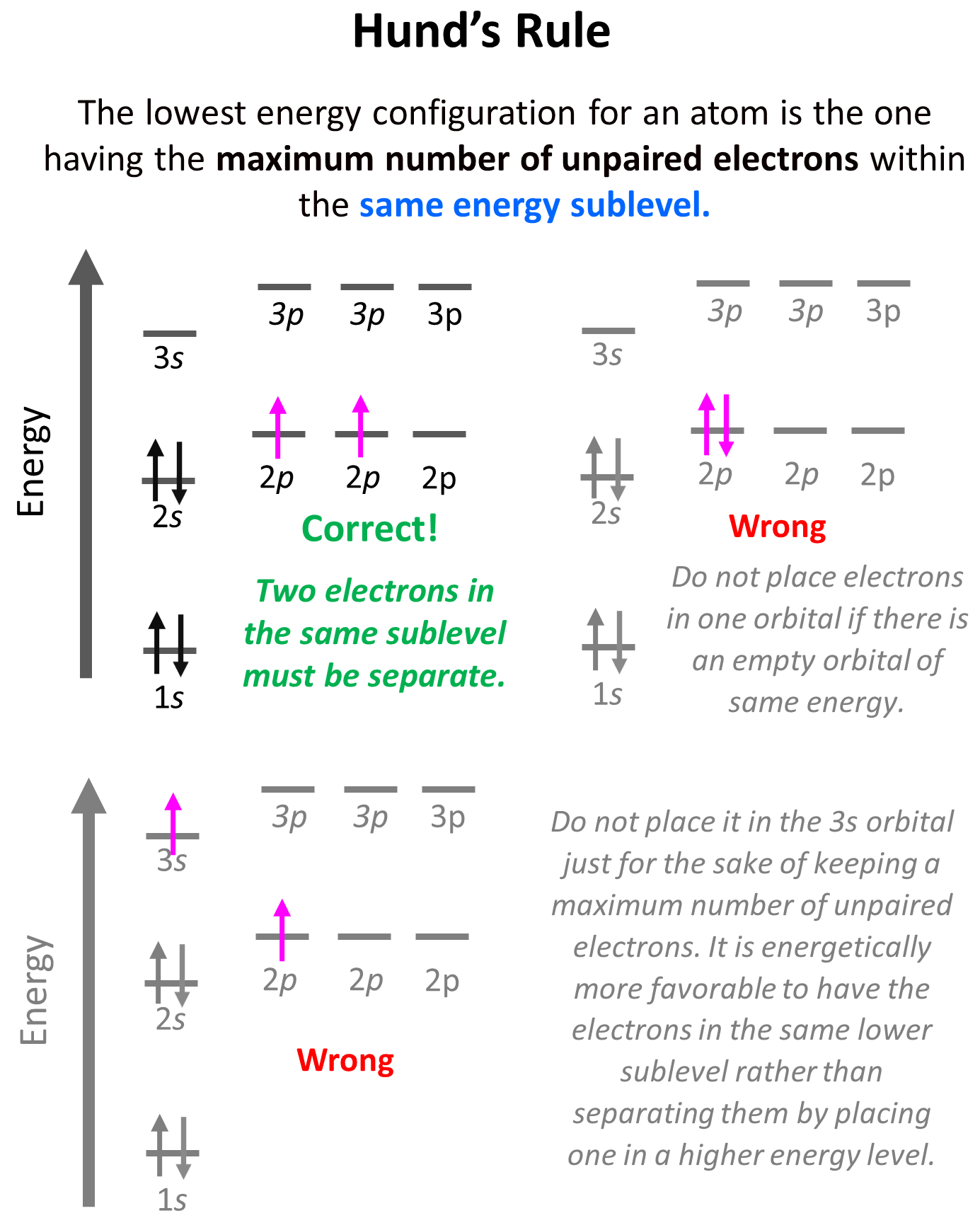Notice that placing the electron unpaired in the 3s orbital is also incorrect because, it is important to mention, that Hund’s rule applies to electrons in the same energy level. Check this article for more information and exception on Hund’s rule, as well the Aufbau’s and Pauli’s exclusion principles.

To summarize, the information given by the quantum numbers, we can say that every set of the four quantum numbers specifies one electron in the atom. The first three describe (n, l, ml) its orbital, and the fourth (ms) describes its spin. Therefore, at one quantum number describing two electrons should be different. This is the Pauli’s exclusion principle which states that no two electrons in an atom can have the same four quantum numbers. So, if the electrons are in the same orbital, they must have the same nlmvalues, and therefore, the only one that can be different is the ms which is the spin of the electron shown by the position of the arrow.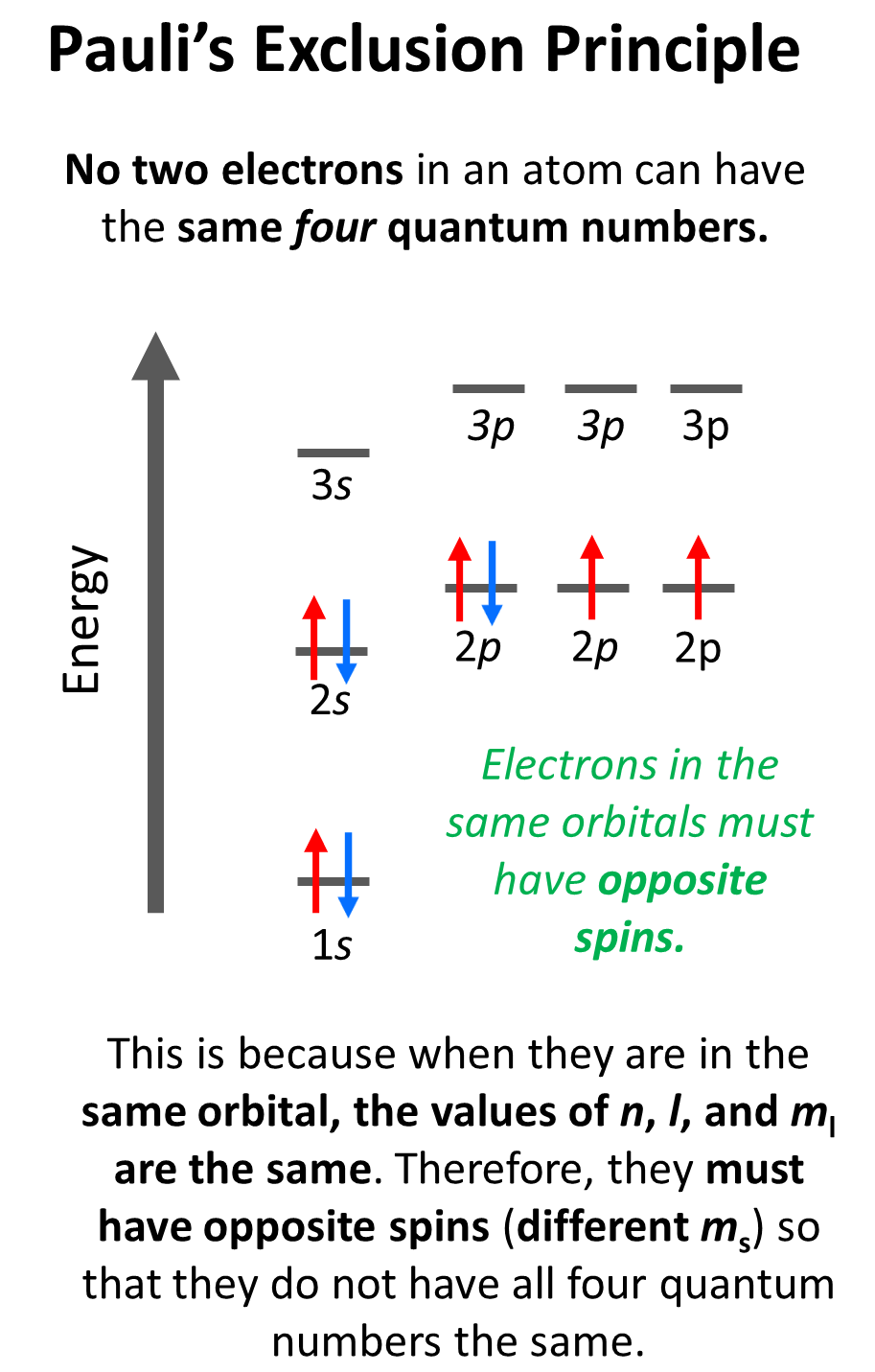For example, for the electrons in a 2p orbital, n = 2, l = 1, and ml = –1, 0, or +1 (doesn’t matter which one because for two electrons in the same orbital, it will be identical). Therefore, the ms must be different (+1/2 or -1/2) in order not to violate Pauli’s exclusion principle.

The values of ms are assigned arbitrarily as we do not know if the first electron is +1/2 or -1/2, however, if we assign it +1/2, then the second must be -1/2 and vice versa. Conventionally though, we put the first arrow pointing up, but it has no scientific evidence, and maybe the other way around.

Let’s do a practice example. What are the values of n, l, ml, and ms for the 3p4 electron?

Solution: The first number is 3 and that is the principal quantum number (n = 3). It is a p orbital, and therefore, l = 1.

For the ml, we need to draw the orbital diagram to fill the electrons one by one and see which p orbital the fourth electron goes to.For the ms, the arrow may be pointing up or down depending on how we drew the first electron. Again, this is arbitrary, however, they must be in opposite directions, and since we assigned +1/2 for the first electron in the first p orbital, the second must be pointing down –  ms = -1/2.

Check this 95-question, Multiple-Choice Quiz on the Electronic Structure of Atoms including questions on properties of light such as wavelength, frequency, energy, quantum numbers, atomic orbitals, electron configurations, and more.

Check Also